Option Calculator and Implied Volatilities

Using data from the web or financial press, evaluate how well the option pricing models explain stock option prices.

This exercise is based on the Option Calculator Module on the Virtual Classroom.  The original numbers for this exercise were obtained closing stock option data from the newspapers.  The Option Calculator allows you to automatically retrieve the latest data from the web.  But by working through this exercise you will learn how to apply the calculator to the real world option markets.

Let us use Intel as an example.  On Dec 8, 1995 , the stock price of Intel at the end of the day was 63 1/4.  January calls and puts were traded for strike prices ranging from 55 to 70, as listed below.

 Strike Price Call Price Put Price 55 9 1/4 7/8 60 5 1/2 1 ¾ 65 2 11/16 4 ¼ 70 1 1/8 7 ¾

To see how well the option pricing models explain this data, we need to know the inputs required by the Option Calculator.  Run the Option Calculator directly from the Virtual Classroom page, to get: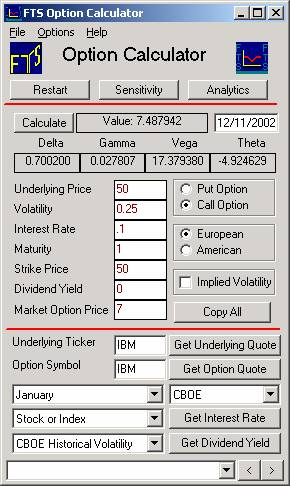Let us start with the Black-Scholes model.  To apply it, we need to know the underlying asset price (which is 63 1/4), the volatility, the risk-free interest rate, the time to maturity, and the strike price.  We do not need to worry about the dividend yield; Intel will not be announcing or paying a dividend between Dec 8 and the option maturity.

Lets start with the maturity date.  Stock options in the US expire on the Saturday following the third Friday of the month.   For current data the Option Calculator will automatically compute the annualized time to maturity for you relative to the current date.  It has a built-in routine to determine expiration dates for options.

For the case of this historical example we have a choice of two ways for entering the time to maturity information.  First is to enter dates directly, and second is to use the FTS Calculator to compute the annualized time to maturity.  We illustrate both ways next.

Maturity + Choice of Entry Formats into the FTS Option Calculator

Current time for example = December 8, 1995

Date of Maturity for the January option 1/20/1996 .

You can enter these two dates directly into the Option Calculator ( US style mm/dd/yyyy).  This is illustrated below in the screen dump titled FTS Option Calculator.  Alternatively, you can use the FTS Calculator to compute the annualized maturity number from two dates, plus the continuous compounding entries for the risk free rate (see the FTS Calculators screen below).

For the case of the time to maturity you can enter the number directly into the FTS Option Calculator (= 0.11780822).  Either form of entry (dates or number) is acceptable to the FTS Option Calculator.

Interest Rate

The Treasury Bill quote for the closest (1/22/1996) maturity is 5.33.  The FTS Calculator (see screen below titled FTS Calculators) will convert this to the continuously compounded rate:

Note:  For both of the above two entries you can use the FTS Calculator if you want to.  Launch from the Virtual Classroom page and enter the appropriate information for settlement and T-bill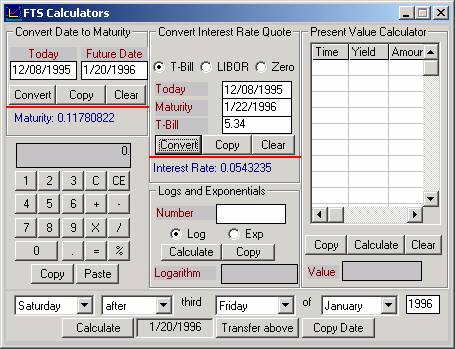For the option calculator we will use 0.0543 as our estimate for the risk free rate.

Spot Price of Underlying Asset

Enter 63.25 in the Underlying Price field.

Strike Price

The first strike price is 55, so enter that into the Strike Price field.

Finally, set the style to American because regular stock options in the US can be exercised at any time.

Click on calculate and your screen should now as follows: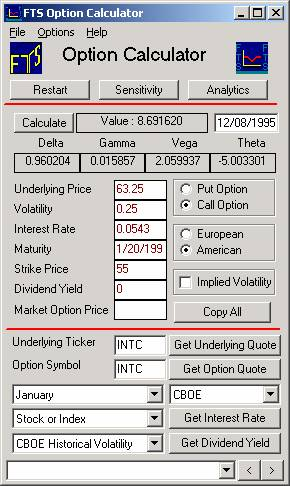The above is the option price with the default volatility, 0.25 (or 25%).  We have not, however, taken any care to assess volatility when estimating the price so far.  So this raised the important question when valuing options --- how do we estimate volatility?  This is important because trading options is exactly equivalent to trading volatility and therefore much care must be taken when entering this input.

The Black-Scholes price above is 8.692 with volatility set at 25%.  The market price is 9 1/4.   Since price is an increasing function of volatility, we can interpret this as saying that our estimate of volatility is too low for Intel.

There are two ways in which we can obtain an estimate of volatility.  One is using historical data, which would require a data set on Intel prices.  The other is to calculate “implied volatility,” i.e., the volatility implicit in the price of the option.

What should we put in for volatility? One answer is to calculate the volatility which makes the Black-Scholes price equal to the market price.

To calculate the volatility implied by the 55-call, click beside Implied Volatility and type in the option’s price (9 1/4) in the new field that appears and click OK.  You should now see: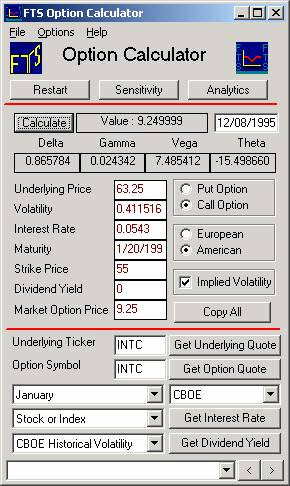The volatility implied by the option price is 41.2%.

Calculating the Implied Volatility for Multiple Options

We can calculate the implied volatilities of all the options.  Of course, if we calculate implied volatilities in this way, then the model will fit the data perfectly.  So how do we tell whether the model provides an accurate description of option prices? The answer comes from the fact the Black Scholes option pricing model requires that the same volatility work for all options.  We can easily test this out by calculating the implied volatilities of all the options.  We simply have to change the strike prices and market prices, and then calculate the implied volatilities for a set of options (see appendix to this lesson for a note on how to automate this when working with your own position).

Consider the basic set of data (let A/P denote American put option and A/C American call option):

 Option Type Asset Price Volatility Interest Rate Maturity Strike Price Dividend Yield Market Price A/P 63.25 .4 .0543 .117808 55 0 7/8 A/P 63.25 .4 .0543 .117808 60 0 1 3/4 A/P 63.25 .4 .0543 .117808 65 0 4 1/4 A/P 63.25 .4 .0543 .117808 70 0 7 3/4 A/C 63.25 .4 .0543 .117808 55 0 9 1/4 A/C 63.25 .4 .0543 .117808 60 0 5 1/2 A/C 63.25 .4 .0543 .117808 65 0 2 11/16 A/C 63.25 .4 .0543 .117808 70 0 1 1/8

It is straightforward to change the strike price, option price and recalculate the implied volatility for puts and calls respectively.  From this we can compute the implied volatilities as follows:

 Option Type Strike Implied Volatility A/P 55 0.4567 A/P 60 0.3840 A/P 65 0.3993 A/P 70 0.4046 A/C 55 0.4116 A/C 60 0.4011 A/C 65 0.3779 A/C 70 0.3722

If you plot the strike price versus the implied volatility for the put options, you get: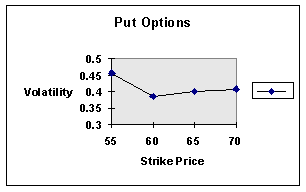Recall that the stock price is 63.25, so the 65-put is closest to the money.  The graph shows evidence of the “volatility smile,” so-named because of the shape of this curve.

For the call, we get: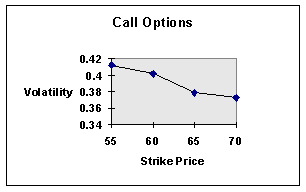You can see that this is less of a “smile” and more of a “smirk.”

The results on implied volatility show that the option pricing model, which assumes a constant volatility across strike prices and across puts and calls, does not fit observed market data precisely.

There are several caveats that must be applied to this conclusion.  First, we are not sure that we have the correct data.  The stock price is taken at the close of the market, but we do not know when  the options traded.  Second, there is typically a bid-ask spread in the market, and the closing prices (of either the stock or the options) do not indicate whether the last trade took place at the bid or the ask.  This makes a difference because the way the model calculates the value of the option is based on the prices at which the stocks and options can be traded.  Third, we have not allowed for other factors such as transaction costs, which will also affect the model.

Using Current Market Data:

Once you have worked through and understood the above historical example you can apply the calculator to current examples.  For Intel the stock symbol is INTC and the option symbol is either NQ or INQ currently depending upon the strike price.  See www.cboe.com Market Quotes (click on Delayed Option Quotes as one way of getting to the symbol directory).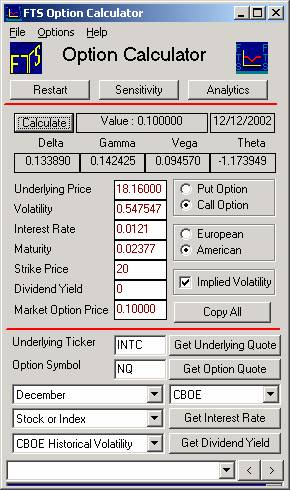Appendix:  Using the Option Portfolio Manager for Larger Positions

When working with your own position involving multiple options, once you master using the Option Calculator (the subject of this lesson), you can also use the Option Portfolio Manager Module.  This module automates much of the tedious work associated with using multiple option quotes.  This module automatically retrieves the latest (delayed) prices from the web for options currently trading on the Chicago Board of Options Exchange (CBOE) or you can link to Excel for other options.  The initial screen for the Option Portfolio Manager appears as follows: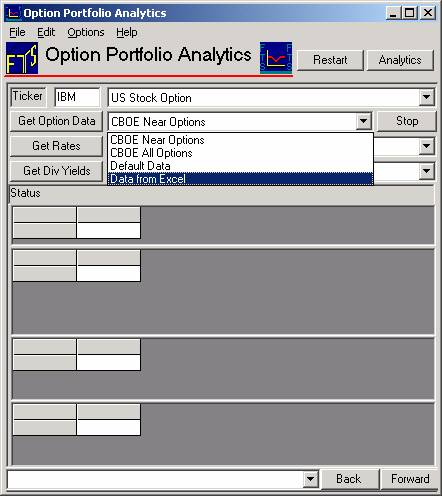You will find that for Option Problems involving few options that the Option Calculator is easier to use.  For problems involving a larger number of options and position considerations then the Option Portfolio Module is preferred.  For example, this module will compute all the implied volatilities of the options in your position automatically, including plotting the Implied Volatility Smile.